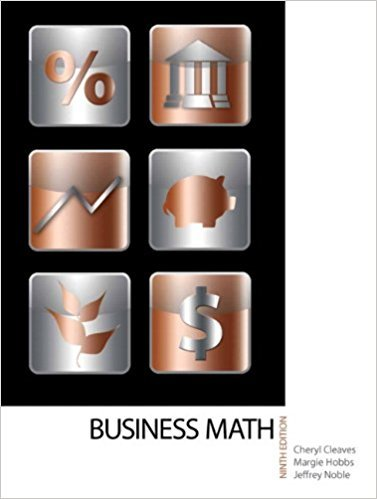×
×

# Solutions for Chapter 6-2: SOLVING PERCENTAGE PROBLEMS## Full solutions for Business Math, | 9th Edition

ISBN: 9780135108178Solutions for Chapter 6-2: SOLVING PERCENTAGE PROBLEMS

Solutions for Chapter 6-2
4 5 0 249 Reviews
10
5
##### ISBN: 9780135108178

Since 34 problems in chapter 6-2: SOLVING PERCENTAGE PROBLEMS have been answered, more than 19424 students have viewed full step-by-step solutions from this chapter. This expansive textbook survival guide covers the following chapters and their solutions. This textbook survival guide was created for the textbook: Business Math, , edition: 9. Business Math, was written by and is associated to the ISBN: 9780135108178. Chapter 6-2: SOLVING PERCENTAGE PROBLEMS includes 34 full step-by-step solutions.

Key Math Terms and definitions covered in this textbook
• Cofactor Cij.

Remove row i and column j; multiply the determinant by (-I)i + j •

• Condition number

cond(A) = c(A) = IIAIlIIA-III = amaxlamin. In Ax = b, the relative change Ilox III Ilx II is less than cond(A) times the relative change Ilob III lib II· Condition numbers measure the sensitivity of the output to change in the input.

• Dimension of vector space

dim(V) = number of vectors in any basis for V.

• Free variable Xi.

Column i has no pivot in elimination. We can give the n - r free variables any values, then Ax = b determines the r pivot variables (if solvable!).

• Full row rank r = m.

Independent rows, at least one solution to Ax = b, column space is all of Rm. Full rank means full column rank or full row rank.

• Jordan form 1 = M- 1 AM.

If A has s independent eigenvectors, its "generalized" eigenvector matrix M gives 1 = diag(lt, ... , 1s). The block his Akh +Nk where Nk has 1 's on diagonall. Each block has one eigenvalue Ak and one eigenvector.

• Left nullspace N (AT).

Nullspace of AT = "left nullspace" of A because y T A = OT.

• Linear combination cv + d w or L C jV j.

• Multiplication Ax

= Xl (column 1) + ... + xn(column n) = combination of columns.

• Multiplier eij.

The pivot row j is multiplied by eij and subtracted from row i to eliminate the i, j entry: eij = (entry to eliminate) / (jth pivot).

• Normal matrix.

If N NT = NT N, then N has orthonormal (complex) eigenvectors.

• Orthogonal matrix Q.

Square matrix with orthonormal columns, so QT = Q-l. Preserves length and angles, IIQxll = IIxll and (QX)T(Qy) = xTy. AlllAI = 1, with orthogonal eigenvectors. Examples: Rotation, reflection, permutation.

• Rank one matrix A = uvT f=. O.

Column and row spaces = lines cu and cv.

• Reduced row echelon form R = rref(A).

Pivots = 1; zeros above and below pivots; the r nonzero rows of R give a basis for the row space of A.

• Row picture of Ax = b.

Each equation gives a plane in Rn; the planes intersect at x.

• Schwarz inequality

Iv·wl < IIvll IIwll.Then IvTAwl2 < (vT Av)(wT Aw) for pos def A.

• Sum V + W of subs paces.

Space of all (v in V) + (w in W). Direct sum: V n W = to}.

• Transpose matrix AT.

Entries AL = Ajj. AT is n by In, AT A is square, symmetric, positive semidefinite. The transposes of AB and A-I are BT AT and (AT)-I.

• Vector space V.

Set of vectors such that all combinations cv + d w remain within V. Eight required rules are given in Section 3.1 for scalars c, d and vectors v, w.

• Vector v in Rn.

Sequence of n real numbers v = (VI, ... , Vn) = point in Rn.

×